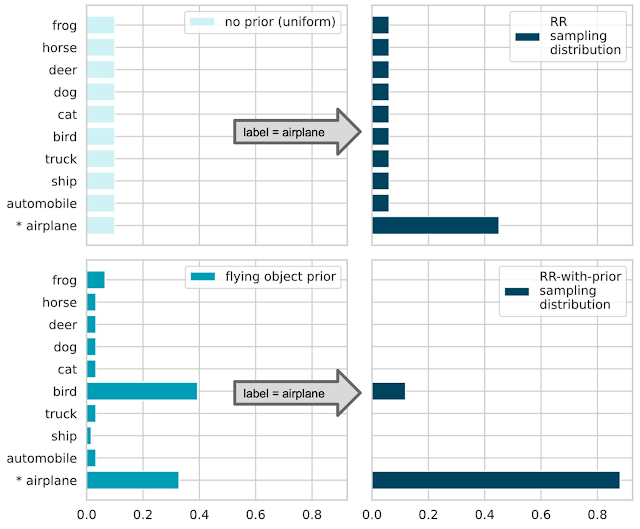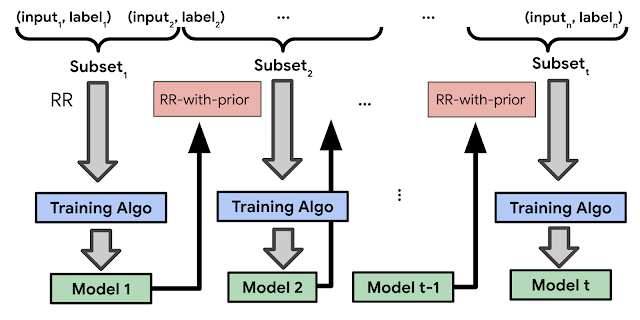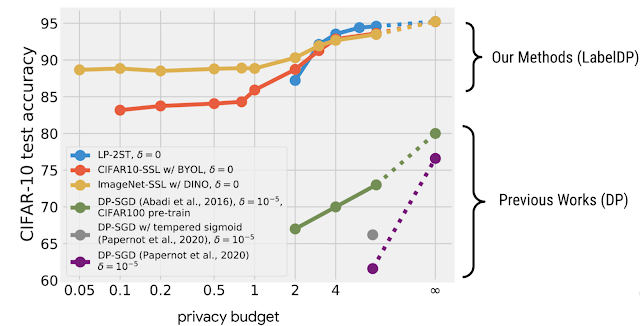# SECRET OF CSS

## NEWS# Deep Learning with Label Differential Privacy

Over the last several years, there has been an increased focus on developing differential privacy (DP) machine learning (ML) algorithms. DP has been the basis of several practical deployments in industry — and has even been employed by the U.S. Census — because it enables the understanding of system and algorithm privacy guarantees. The underlying assumption of DP is that changing a single user’s contribution to an algorithm should not significantly change its output distribution.

In the standard supervised learning setting, a model is trained to make a prediction of the label for each input given a training set of example pairs {[input1,label1], …, [inputn, labeln]}. In the case of deep learning, previous work introduced a DP training framework, DP-SGD, that was integrated into TensorFlow and PyTorch. DP-SGD protects the privacy of each example pair [input, label] by adding noise to the stochastic gradient descent (SGD) training algorithm. Yet despite extensive efforts, in most cases, the accuracy of models trained with DP-SGD remains significantly lower than that of non-private models.

DP algorithms include a privacy budget, ε, which quantifies the worst-case privacy loss for each user. Specifically, ε reflects how much the probability of any particular output of a DP algorithm can change if one replaces any example of the training set with an arbitrarily different one. So, a smaller ε corresponds to better privacy, as the algorithm is more indifferent to changes of a single example. However, since smaller ε tends to hurt model utility more, it is not uncommon to consider ε up to 8 in deep learning applications. Notably, for the widely used multiclass image classification dataset, CIFAR-10, the highest reported accuracy (without pre-training) for DP models with ε = 3 is 69.3%, a result that relies on handcrafted visual features. In contrast, non-private scenarios (ε = ∞) with learned features have shown to achieve >95% accuracy while using modern neural network architectures. This performance gap remains a roadblock for many real-world applications to adopt DP. Moreover, despite recent advances, DP-SGD often comes with increased computation and memory overhead due to slower convergence and the need to compute the norm of the per-example gradient.

In “Deep Learning with Label Differential Privacy”, presented at NeurIPS 2021, we consider a more relaxed, but important, special case called label differential privacy (LabelDP), where we assume the inputs (input1, …, inputn) are public, and only the privacy of the training labels (label1, …, labeln) needs to be protected. With this relaxed guarantee, we can design novel algorithms that utilize a prior understanding of the labels to improve the model utility. We demonstrate that LabelDP achieves 20% higher accuracy than DP-SGD on the CIFAR-10 dataset. Our results across multiple tasks confirm that LabelDP could significantly narrow the performance gap between private models and their non-private counterparts, mitigating the challenges in real world applications. We also present a multi-stage algorithm for training deep neural networks with LabelDP. Finally, we are excited to release the code for this multi-stage training algorithm.

LabelDP

The notion of LabelDP has been studied in the Probably Approximately Correct (PAC) learning setting, and captures several practical scenarios. Examples include: (i) computational advertising, where impressions are known to the advertiser and thus considered non-sensitive, but conversions reveal user interest and are thus private; (ii) recommendation systems, where the choices are known to a streaming service provider, but the user ratings are considered sensitive; and (iii) user surveys and analytics, where demographic information (e.g., age, gender) is non-sensitive, but income is sensitive.

We make several key observations in this scenario. (i) When only the labels need to be protected, much simpler algorithms can be applied for data preprocessing to achieve LabelDP without any modifications to the existing deep learning training pipeline. For example, the classic Randomized Response (RR) algorithm, designed to eliminate evasive answer biases in survey aggregation, achieves LabelDP by simply flipping the label to a random one with a probability that depends on ε. (ii) Conditioned on the (public) input, we can compute a prior probability distribution, which provides a prior belief of the likelihood of the class labels for the given input. With a novel variant of RR, RR-with-prior, we can incorporate prior information to reduce the label noise while maintaining the same privacy guarantee as classical RR.

The figure below illustrates how RR-with-prior works. Assume a model is built to classify an input image into 10 categories. Consider a training example with the label “airplane”. To guarantee LabelDP, classical RR returns a random label sampled according to a given distribution (see the top-right panel of the figure below). The smaller the targeted privacy budget ε is, the larger the probability of sampling an incorrect label has to be. Now assume we have a prior probability showing that the given input is “likely an object that flies” (lower left panel). With the prior, RR-with-prior will discard all labels with small prior and only sample from the remaining labels. By dropping these unlikely labels, the probability of returning the correct label is significantly increased, while maintaining the same privacy budget ε (lower right panel).Randomized response: If no prior information is given (top-left), all classes are sampled with equal probability. The probability of sampling the true class (P[airplane] ≈ 0.5) is higher if the privacy budget is higher (top-right). RR-with-prior: Assuming a prior distribution (bottom-left), unlikely classes are “suppressed” from the sampling distribution (bottom-right). So the probability of sampling the true class (P[airplane] ≈ 0.9) is increased under the same privacy budget.

A Multi-stage Training Algorithm

Based on the RR-with-prior observations, we present a multi-stage algorithm for training deep neural networks with LabelDP. First, the training set is randomly partitioned into multiple subsets. An initial model is then trained on the first subset using classical RR. Finally, the algorithm divides the data into multiple parts, and at each stage, a single part is used to train the model. The labels are produced using RR-with-prior, and the priors are based on the prediction of the model trained so far.An illustration of the multi-stage training algorithm. The training set is partitioned into t disjoint subsets. An initial model is trained on the first subset using classical RR. Then the trained model is used to provide prior predictions in the RR-with-prior step and in the training of the later stages.

Results

We benchmark the multi-stage training algorithm’s empirical performance on multiple datasets, domains, and architectures. On the CIFAR-10 multi-class classification task for the same privacy budget ε, the multi-stage training algorithm (blue in the figure below) guaranteeing LabelDP achieves 20% higher accuracy than DP-SGD. We emphasize that LabelDP protects only the labels while DP-SGD protects both the inputs and labels, so this is not a strictly fair comparison. Nonetheless, this result demonstrates that for specific application scenarios where only the labels need to be protected, LabelDP could lead to significant improvements in the model utility while narrowing the performance gap between private models and public baselines.Comparison of the model utility (test accuracy) of different algorithms under different privacy budgets.

In some domains, prior knowledge is naturally available or can be built using publicly available data only. For example, many machine learning systems have historical models which could be evaluated on new data to provide label priors. In domains where unsupervised or self-supervised learning algorithms work well, priors could also be built from models pre-trained on unlabeled (therefore public with respect to LabelDP) data. Specifically, we demonstrate two self-supervised learning algorithms in our CIFAR-10 evaluation (orange and green traces in the figure above). We use self-supervised learning models to compute representations for the training examples and run k-means clustering on the representations. Then, we spend a small amount of privacy budget (ε ≤ 0.05) to query a histogram of the label distribution of each cluster and use that as the label prior for the points in each cluster. This prior significantly boosts the model utility in the low privacy budget regime (ε < 1).

Similar observations hold across multiple datasets such as MNIST, Fashion-MNIST and non-vision domains, such as the MovieLens-1M movie rating task. Please see our paper for the full report on the empirical results.

The empirical results suggest that protecting the privacy of the labels can be significantly easier than protecting the privacy of both the inputs and labels. This can also be mathematically proven under specific settings. In particular, we can show that for convex stochastic optimization, the sample complexity of algorithms privatizing the labels is much smaller than that of algorithms privatizing both labels and inputs. In other words, to achieve the same level of model utility under the same privacy budget, LabelDP requires fewer training examples.

Conclusion

We demonstrated that both empirical and theoretical results suggest that LabelDP is a promising relaxation of the full DP guarantee. In applications where the privacy of the inputs does not need to be protected, LabelDP could reduce the performance gap between a private model and the non-private baseline. For future work, we plan to design better LabelDP algorithms for other tasks beyond multi-class classification. We hope that the release of the multi-stage training algorithm code provides researchers with a useful resource for DP research.

Acknowledgements

This work was carried out in collaboration with Badih Ghazi, Noah Golowich, and Ravi Kumar. We also thank Sami Torbey for valuable feedback on our work.